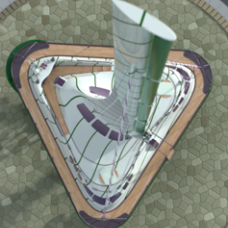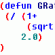# Add Dimensions to Polyline in VBA## Recommended PostsI started a routine to add dimensions to polyline...

The problem is that is created over the line and I want to create it separated from the line, If some one can help me with this will be awesome...

thanks,

```Sub add_dim_polyline()
Dim polyCoords As Variant
Dim getPoint As Variant
Dim a As Integer
Dim polyCoordBound As Integer
Dim stPoint(2) As Double
Dim ePoint(2) As Double
Dim sectionAngle As Double
Dim textCoords As Variant
Dim polyDist As Double
Dim x1, x2, y1, y2 As Double
ThisDrawing.Utility.GetEntity ThePolyline, getPoint, "Select an object"
polyCoords = ThePolyline.Coordinates
polyCoordBound = UBound(polyCoords)

For a = 0 To polyCoordBound - 1 Step 2
If a = polyCoordBound - 1 Then
stPoint(0) = polyCoords(a)
stPoint(1) = polyCoords(a + 1)
stPoint(2) = 0
ePoint(0) = polyCoords(0)
ePoint(1) = polyCoords(1)
ePoint(2) = 0
Else
stPoint(0) = polyCoords(a)
stPoint(1) = polyCoords(a + 1)
stPoint(2) = 0
ePoint(0) = polyCoords(a + 2)
ePoint(1) = polyCoords(a + 3)
ePoint(2) = 0

End If
x1 = stPoint(0): x2 = ePoint(0)
y1 = stPoint(1): y2 = ePoint(1)
polyDist = Sqr(((x1 - x2) ^ 2) + ((y1 - y2) ^ 2))
sectionAngle = ThisDrawing.Utility.AngleFromXAxis(stPoint, ePoint)
textCoords = ThisDrawing.Utility.PolarPoint(stPoint, sectionAngle, polyDist / 2)
Set objDimAligned = ThisDrawing.ModelSpace.AddDimAligned(stPoint, ePoint, textCoords)

Next a
End Sub
```

##### Share on other sitesPerhaps something like the addition shown here.

```sectionAngle = ThisDrawing.Utility.AngleFromXAxis(stPoint, ePoint)
textCoords = ThisDrawing.Utility.PolarPoint(stPoint, sectionAngle, polyDist / 2)
textCoords = ThisDrawing.Utility.PolarPoint(textCoords, sectionAngle + 1.57, 1#) 'Rotate PI/2
Set objDimAligned = ThisDrawing.ModelSpace.AddDimAligned(stPoint, ePoint, textCoords)```

##### Share on other sites```Sub add_dim_polyline()
Dim polyCoords As Variant
Dim getPoint As Variant
Dim a As Integer
Dim polyCoordBound As Integer
Dim stPoint(2) As Double
Dim ePoint(2) As Double
Dim sectionAngle As Double
Dim textCoords As Variant
Dim polyDist As Double
Dim x1, x2, y1, y2 As Double
Dim textAngle As Double
ThisDrawing.Utility.GetEntity ThePolyline, getPoint, "Select an object"
polyCoords = ThePolyline.Coordinates
polyCoordBound = UBound(polyCoords)

For a = 0 To polyCoordBound - 2 Step 2
stPoint(0) = polyCoords(a)
stPoint(1) = polyCoords(a + 1)
stPoint(2) = 0
ePoint(0) = polyCoords(a + 2)
ePoint(1) = polyCoords(a + 3)
ePoint(2) = 0

x1 = stPoint(0): x2 = ePoint(0)
y1 = stPoint(1): y2 = ePoint(1)
polyDist = Sqr(((x1 - x2) ^ 2) + ((y1 - y2) ^ 2))
sectionAngle = ThisDrawing.Utility.AngleFromXAxis(stPoint, ePoint)
textAngle = sectionAngle + 1.57
textCoords = ThisDrawing.Utility.PolarPoint(stPoint, sectionAngle + 1.57, 1#)
Set objDimAligned = ThisDrawing.ModelSpace.AddDimAligned(stPoint, ePoint, textCoords)

Next a
End Sub```

##### Share on other sitesHeyy, I just saw this post, can someone of you tell me what is this VBA about?? When drawing polyline it shows and writes distance up to line or..??

##### Share on other sitesActually, the structure of the routine is to select a polyline and automatically add dimensions to it.

##### Share on other sitesSounds good, I have something similiar lisp, you select polyline and it puts dimension on it (between two dots on pline, and so on along line), how you use VBA?? never done that so I'm curious...in few words if you have time to write how to start it..##### Share on other sitesSounds good, I have something similiar lisp, you select polyline and it puts dimension on it (between two dots on pline, and so on along line), how you use VBA?? never done that so I'm curious...in few words if you have time to write how to start it..I'm still not permitted to stamp addresses but google "autocad vba" and will appear some hyperpics dot com page with an introduction of vba

##### Share on other sitesI'm still not permitted to stamp addresses but google "autocad vba" and will appear some hyperpics dot com page with an introduction of vba

Ok, understand...Thx man!!!##### Share on other sites

• 4 years later...I started a routine to add dimensions to polyline...

The problem is that is created over the line and I want to create it separated from the line, If some one can help me with this will be awesome...

thanks,

```Sub add_dim_polyline()
Dim polyCoords As Variant
Dim getPoint As Variant
Dim a As Integer
Dim polyCoordBound As Integer
Dim stPoint(2) As Double
Dim ePoint(2) As Double
Dim sectionAngle As Double
Dim textCoords As Variant
Dim polyDist As Double
Dim x1, x2, y1, y2 As Double
ThisDrawing.Utility.GetEntity ThePolyline, getPoint, "Select an object"  <--???
polyCoords = ThePolyline.Coordinates
polyCoordBound = UBound(polyCoords)

For a = 0 To polyCoordBound - 1 Step 2
If a = polyCoordBound - 1 Then
stPoint(0) = polyCoords(a)
stPoint(1) = polyCoords(a + 1)
stPoint(2) = 0
ePoint(0) = polyCoords(0)
ePoint(1) = polyCoords(1)
ePoint(2) = 0
Else
stPoint(0) = polyCoords(a)
stPoint(1) = polyCoords(a + 1)
stPoint(2) = 0
ePoint(0) = polyCoords(a + 2)
ePoint(1) = polyCoords(a + 3)
ePoint(2) = 0

End If
x1 = stPoint(0): x2 = ePoint(0)
y1 = stPoint(1): y2 = ePoint(1)
polyDist = Sqr(((x1 - x2) ^ 2) + ((y1 - y2) ^ 2))
sectionAngle = ThisDrawing.Utility.AngleFromXAxis(stPoint, ePoint)
textCoords = ThisDrawing.Utility.PolarPoint(stPoint, sectionAngle, polyDist / 2)
Set objDimAligned = ThisDrawing.ModelSpace.AddDimAligned(stPoint, ePoint, textCoords)

Next a
End Sub
```

this code can''t work,
##### Share on other sitesWelcome to the Forum, Iyant!

Can you be more specific, please? How you loaded/called the code? Did you got any error message? And last, but not least, in which version did you attempted to test it?

##### Share on other sites

• 3 weeks later...MSasu, i made polyline from data excel and need to show angular dimension, but always got error message

##### Share on other sitesIt would be useful to post the error you got to allow someone to provide you with an answer.

## Join the conversation

You can post now and register later. If you have an account, sign in now to post with your account.
Note: Your post will require moderator approval before it will be visible.Unfortunately, your content contains terms that we do not allow. Please edit your content to remove the highlighted words below.

×   Pasted as rich text.   Restore formatting

Only 75 emoji are allowed.